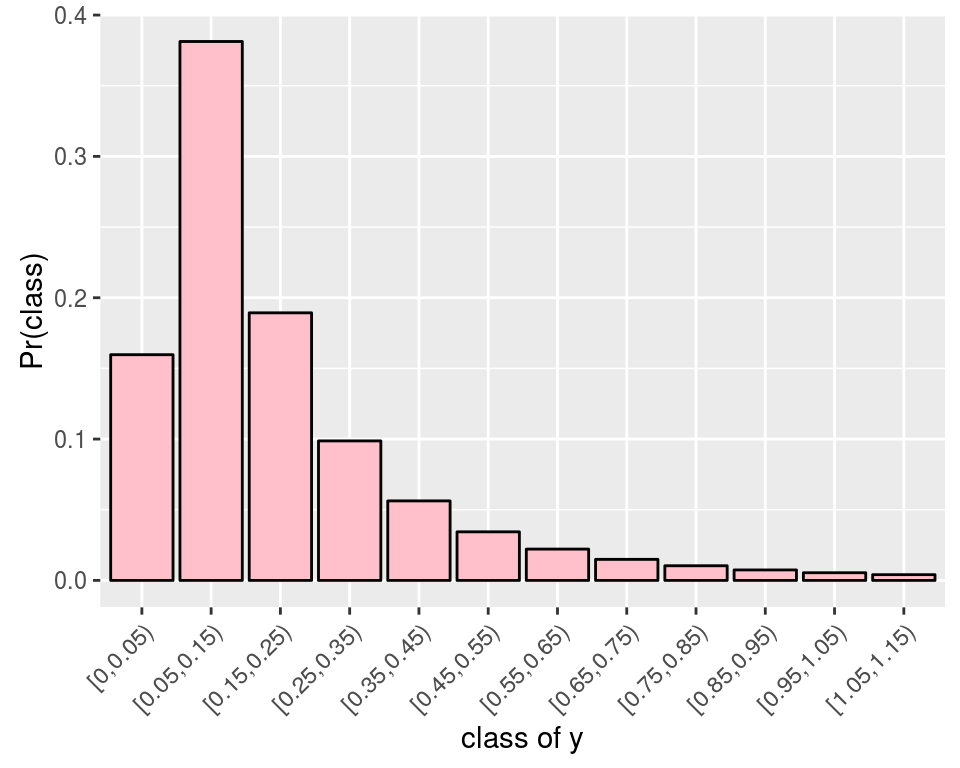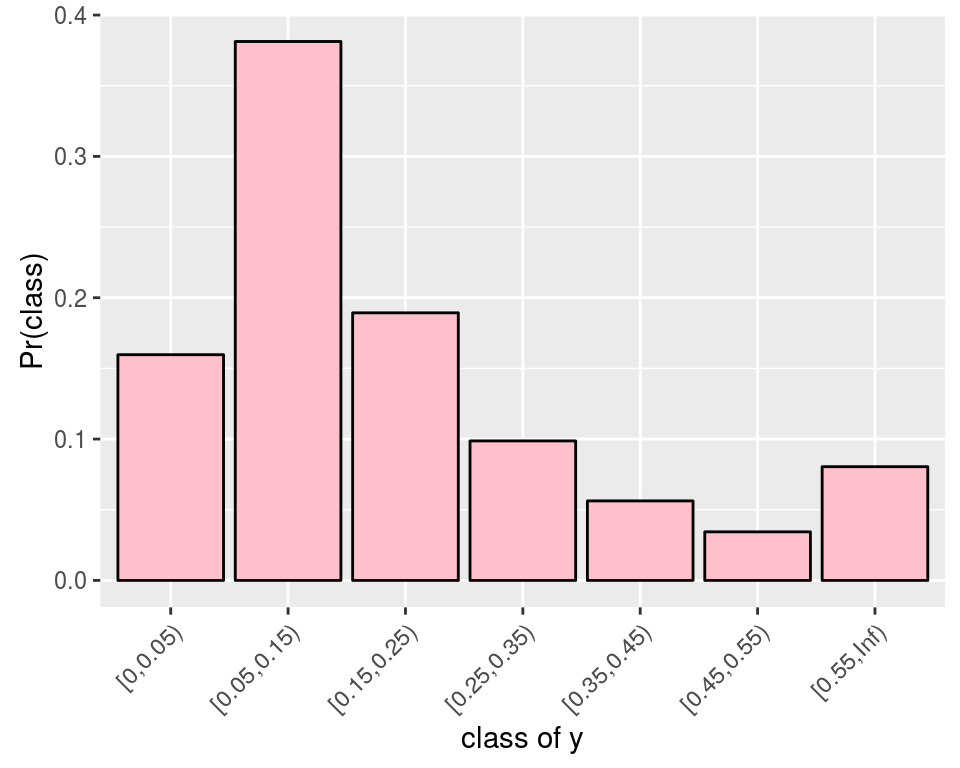1. Dealing with rounded data

2016-03-24
Source

Real “continuous” data are always rounded. For instance, I already had to deal with these data:

##    factor1 factor2   y
## 1       A1      B1 0.1
## 2       A1      B1 0.1
## 3       A1      B1 0.1
## 4       A1      B1 0.1
## 5       A1      B1 0.1
## 6       A1      B1 0.1
## 7       A1      B1 0.3
## 8       A1      B1 0.3
## 9       A1      B1 0.1
## 10      A1      B2 0.2
## 11      A1      B2 0.1
## 12      A1      B2 0.1
## 13      A1      B2 0.1
## 14      A1      B2 0.0
## 15      A1      B2 0.0

These data were recorded by a measurement device with one decimal precision. Thus, a value of $0.1$ actually means the value lies between $0.05$ and $0.15$. A value of $0$ actually means the value lies beteen $0$ and $0.05$ (these are nonnegative measurements).

In fact these are intervals data:

dat1 <- transform(dat, low = pmax(0,y-0.05), up = y+0.05)
##    factor1 factor2   y  low   up
## 1       A1      B1 0.1 0.05 0.15
## 2       A1      B1 0.1 0.05 0.15
## 3       A1      B1 0.1 0.05 0.15
## 4       A1      B1 0.1 0.05 0.15
## 5       A1      B1 0.1 0.05 0.15
## 6       A1      B1 0.1 0.05 0.15
## 7       A1      B1 0.3 0.25 0.35
## 8       A1      B1 0.3 0.25 0.35
## 9       A1      B1 0.1 0.05 0.15
## 10      A1      B2 0.2 0.15 0.25
## 11      A1      B2 0.1 0.05 0.15
## 12      A1      B2 0.1 0.05 0.15
## 13      A1      B2 0.1 0.05 0.15
## 14      A1      B2 0.0 0.00 0.05
## 15      A1      B2 0.0 0.00 0.05

Thus, assuming for instance that the true values of the measurements follow a log-normal distribution:then one should use a rounded log-normal distribution to model the data:By the way, one would get a problem if one intended to fit a log-normal distribution to the y values, because there are some zero values.

Using the grouped package

One way to deal with this issue is to use the grouped R package. It allows to fit linear regression models to grouped data. It is very easy to use:

library(grouped)
fit <- grouped(cbind(low, up) ~  factor1*factor2, link="log", data=dat1)
summary(fit)
##
## Call:
## grouped(formula = cbind(low, up) ~ factor1 * factor2, link = "log",     data = dat1)
##
## Model Summary:
##  log.Lik    AIC     BIC
##  -44.711 99.421 107.739
##
## Coefficients:
##                     Esimate Std.error t.value p.value
## (Intercept)          -2.111     0.217   -9.73  <0.001
## factor1A2             0.551     0.289    1.90   0.065
## factor2B2            -0.574     0.301   -1.90   0.065
## factor1A2:factor2B2  -0.551     0.414   -1.33   0.193
##
## Random-Effect:
## sigma 0.579    0.0821        log-normal
##
## Optimization:
## Convergence: 0
##  Outer iter: 1

The grouped package provides confidence intervals “$\textrm{estimate}\pm z_{1-\frac{\alpha}{2}}\textrm{stderr}$”:

confint(fit)
##                           2.5 %     97.5 %
## (Intercept)         -2.53560037 -1.6854123
## factor1A2           -0.01637233  1.1174238
## factor2B2           -1.16437699  0.0168808
## factor1A2:factor2B2 -1.36285840  0.2617881

This method to get confidence intervals is an asymptotic one, and they are possibly too short for small sample sizes.

A Bayesian solution using STAN

With STAN, one can assign a rounded log-normal distribution to the observations with the help of the categorical distribution.

We use the cut function to create the classes of the measurements:

cuts <- c(0, seq(0.05, max(dat$y)+0.1, by=0.1), Inf) dat2 <- transform(dat, class=cut(y, cuts, right=FALSE)) summary(dat2) ## factor1 factor2 y class ## A1:19 B1:19 Min. :0.0000 [0,0.05) : 6 ## A2:20 B2:20 1st Qu.:0.1000 [0.05,0.15):23 ## Median :0.1000 [0.15,0.25): 3 ## Mean :0.1385 [0.25,0.35): 4 ## 3rd Qu.:0.1500 [0.35,0.45): 2 ## Max. :0.5000 [0.45,0.55): 1 ## [0.55,Inf) : 0 There is no value beyond $0.55$, hence we will fit such a categorical distribution:where the probabilities of the classes are given by the cdf of the log-normal distribution: $\Pr\bigl([a,b)\bigr) = \Phi\left(\frac{\log(b)-\mu}{\sigma}\right) - \Phi\left(\frac{\log(a)-\mu}{\sigma} \right).$ The support of the categorical distribution in STAN is $1$, $2$, $\ldots$, $K$, so we have to encode each class by an integer: dat2 <- transform(dat2, ycat=as.integer(class)) head(dat2, 15) ## factor1 factor2 y class ycat ## 1 A1 B1 0.1 [0.05,0.15) 2 ## 2 A1 B1 0.1 [0.05,0.15) 2 ## 3 A1 B1 0.1 [0.05,0.15) 2 ## 4 A1 B1 0.1 [0.05,0.15) 2 ## 5 A1 B1 0.1 [0.05,0.15) 2 ## 6 A1 B1 0.1 [0.05,0.15) 2 ## 7 A1 B1 0.3 [0.25,0.35) 4 ## 8 A1 B1 0.3 [0.25,0.35) 4 ## 9 A1 B1 0.1 [0.05,0.15) 2 ## 10 A1 B2 0.2 [0.15,0.25) 3 ## 11 A1 B2 0.1 [0.05,0.15) 2 ## 12 A1 B2 0.1 [0.05,0.15) 2 ## 13 A1 B2 0.1 [0.05,0.15) 2 ## 14 A1 B2 0.0 [0,0.05) 1 ## 15 A1 B2 0.0 [0,0.05) 1 Now we write and compile the STAN model: library(rstan) rstan_options(auto_write = TRUE) options(mc.cores = parallel::detectCores()) stancode <- " data { int<lower=1> N; // number of observations int<lower=1> ycat[N]; // observations int<lower=1> P; // number of parameters matrix[N,P] X; // model matrix int<lower=1> K; // number of categories vector[K-1] cuts; // the cuts 0.05, 0.15, ..., 0.55 } parameters { vector[P] beta; real<lower=0> sigma; } transformed parameters { vector[N] mu; simplex[K] p[N]; mu <- X*beta; for(i in 1:N){ p[i] <- Phi((log(cuts)-mu[i])/sigma); for(j in 2:(K-1)){ p[i][j] <- Phi((log(cuts[j])-mu[i])/sigma) - Phi((log(cuts[j-1])-mu[i])/sigma); } p[i][K] <- 1.0 - sum(p[i][1:(K-1)]); } } model { for(i in 1:N) ycat[i] ~ categorical(p[i]); beta ~ normal(0, 20); // prior on the regression coefficients sigma ~ cauchy(0, 5); // prior on the standard deviation } " # Compilation stanmodel <- stan_model(model_code = stancode, model_name="rounded 2-way ANOVA") And we run the STAN sampler: # Stan data K <- length(cuts)-1 X <- model.matrix(~factor1*factor2, data=dat2) standata <- list(ycat=dat2$ycat, N=nrow(dat2), K=K, cuts=cuts[2:K], X=X, P=ncol(X))
# Run Stan
samples <- sampling(stanmodel, data = standata,
iter = 11000, warmup = 1000, chains = 4)
# Outputs
library(coda)
codasamples <- do.call(mcmc.list,
plyr::alply(rstan::extract(samples, permuted=FALSE,
pars=c("beta", "sigma")), 2, mcmc))
summary(codasamples)
##
## Iterations = 1:10000
## Thinning interval = 1
## Number of chains = 4
## Sample size per chain = 10000
##
## 1. Empirical mean and standard deviation for each variable,
##    plus standard error of the mean:
##
##            Mean     SD  Naive SE Time-series SE
## beta -2.1228 0.2411 0.0012055      0.0022252
## beta  0.5479 0.3240 0.0016199      0.0029783
## beta -0.5780 0.3369 0.0016843      0.0031244
## beta -0.5470 0.4629 0.0023146      0.0042449
## sigma    0.6537 0.1032 0.0005162      0.0008849
##
## 2. Quantiles for each variable:
##
##             2.5%     25%     50%     75%    97.5%
## beta -2.60292 -2.2815 -2.1209 -1.9626 -1.65339
## beta -0.09319  0.3348  0.5483  0.7637  1.18396
## beta -1.24907 -0.7990 -0.5791 -0.3557  0.08303
## beta -1.46832 -0.8486 -0.5450 -0.2444  0.37006
## sigma    0.48257  0.5813  0.6429  0.7145  0.88888

The estimates of the regression parameters are almost the same as the ones provided by the grouped package, and confidence intervals are a bit larger. The estimate of $\sigma$ is a bit different.

Of course, the major advantage of the Bayesian way is that it can be used for any parametric model, not only the linear regression models.